UPSC  >  Practice Test: Blood Relations - 1

# Practice Test: Blood Relations - 1 - UPSC

Test Description

## 10 Questions MCQ Test CSAT Preparation - Practice Test: Blood Relations - 1

Practice Test: Blood Relations - 1 for UPSC 2023 is part of CSAT Preparation preparation. The Practice Test: Blood Relations - 1 questions and answers have been prepared according to the UPSC exam syllabus.The Practice Test: Blood Relations - 1 MCQs are made for UPSC 2023 Exam. Find important definitions, questions, notes, meanings, examples, exercises, MCQs and online tests for Practice Test: Blood Relations - 1 below.
Solutions of Practice Test: Blood Relations - 1 questions in English are available as part of our CSAT Preparation for UPSC & Practice Test: Blood Relations - 1 solutions in Hindi for CSAT Preparation course. Download more important topics, notes, lectures and mock test series for UPSC Exam by signing up for free. Attempt Practice Test: Blood Relations - 1 | 10 questions in 20 minutes | Mock test for UPSC preparation | Free important questions MCQ to study CSAT Preparation for UPSC Exam | Download free PDF with solutions
 1 Crore+ students have signed up on EduRev. Have you?
Practice Test: Blood Relations - 1 - Question 1

### Direction: Study the following information carefully to answer the given questions: If 'A () B' means 'B is the mother of A' If 'A of B' means 'B is the father of A' If 'A ÷ B' means 'B is the sister of A' If 'A × B' means 'B is the brother of A' If 'A + B' means 'B is the daughter of A' If 'A - B' means 'B is the son of A' Q. If 'T - P ÷ Q' is given, then how is Q related to T?

Detailed Solution for Practice Test: Blood Relations - 1 - Question 1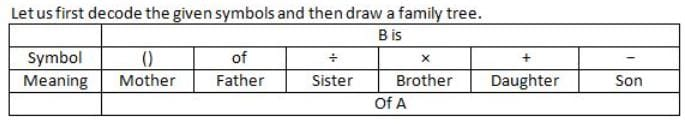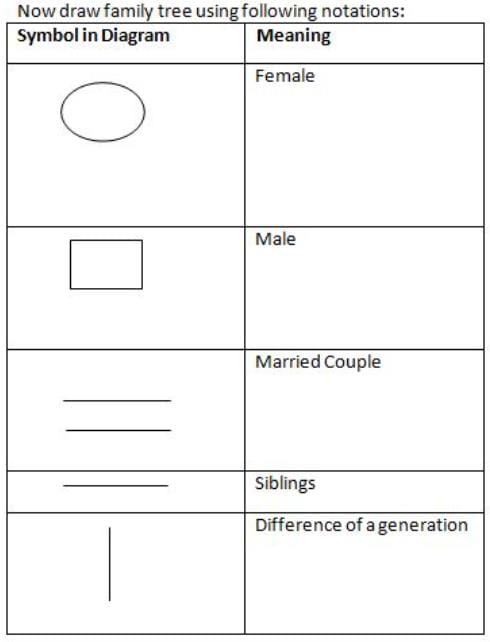'T - P ÷ Q' means P is son of T, Q is sister of P.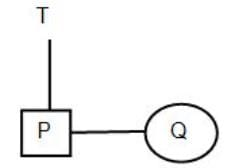Hence, Q is daughter of T.

Practice Test: Blood Relations - 1 - Question 2

### Direction: Study the following information carefully to answer the given questions: If 'A () B' means 'B is the mother of A' If 'A of B' means 'B is the father of A' If 'A ÷ B' means 'B is the sister of A' If 'A × B' means 'B is the brother of A' If 'A + B' means 'B is the daughter of A' If 'A - B' means 'B is the son of A' Q. If 'P of Q + R' is given, then which of the following is true?

Detailed Solution for Practice Test: Blood Relations - 1 - Question 2

'P of Q + R' means Q is the father of P, R is the daughter of Q.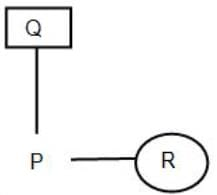Hence, R is the sister of P.

Practice Test: Blood Relations - 1 - Question 3

### Direction: Study the following information carefully to answer the given questions: If 'A () B' means 'B is the mother of A' If 'A of B' means 'B is the father of A' If 'A ÷ B' means 'B is the sister of A' If 'A × B' means 'B is the brother of A' If 'A + B' means 'B is the daughter of A' If 'A - B' means 'B is the son of A' Q. What does 'L () M ÷ N' means?

Detailed Solution for Practice Test: Blood Relations - 1 - Question 3

'L() M ÷ N' means M is mother of L, N is sister of M.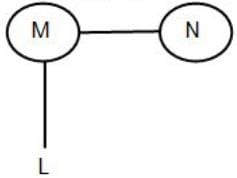Hence, M and N are both sisters.

Practice Test: Blood Relations - 1 - Question 4

Direction: Study the following information carefully to answer the given questions:

If 'A () B' means 'B is the mother of A'

If 'A of B' means 'B is the father of A'

If 'A ÷ B' means 'B is the sister of A'

If 'A × B' means 'B is the brother of A'

If 'A + B' means 'B is the daughter of A'

If 'A - B' means 'B is the son of A'

Q. If 'Y x Z () K' is given, how is Z related to K?

Detailed Solution for Practice Test: Blood Relations - 1 - Question 4

'Yx Z () K' means Z is the brother of Y, K is the mother of Z.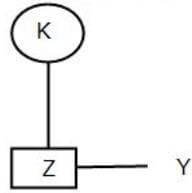Hence, Z is the son of K.

Practice Test: Blood Relations - 1 - Question 5

Direction: Study the following information carefully to answer the given questions:

If 'A () B' means 'B is the mother of A'

If 'A of B' means 'B is the father of A'

If 'A ÷ B' means 'B is the sister of A'

If 'A × B' means 'B is the brother of A'

If 'A + B' means 'B is the daughter of A'

If 'A - B' means 'B is the son of A'

Q. If 'S x J + T' is given then which of the following is true?

Detailed Solution for Practice Test: Blood Relations - 1 - Question 5

'S x J+T' means J is the brother of S, T is the daughter of J.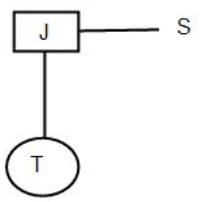Hence, T is the niece of S.

Practice Test: Blood Relations - 1 - Question 6

Directions: Study the following information carefully to answer the given Questions:

P^Q-P is the child of Q
P!Q-P is the parent of Q
P*Q - P is elder to Q
P#Q-P is younger to Q
P@Q-P is brother of Q
P&Q - P is wife of Q
P+Q-P is sister-in-law of Q

Q. If G! A^T+J&O@LP! G, then how is J related to A?

Detailed Solution for Practice Test: Blood Relations - 1 - Question 6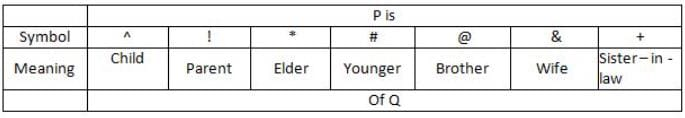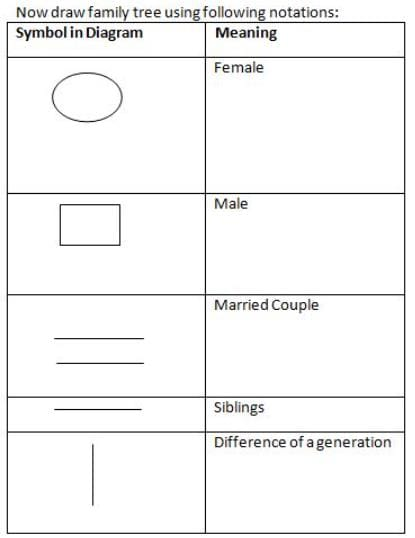G!A^T+J&O @L^P! G: G is parent of A, A is child of T, T is sister-in-law of J, J is wife of O, O is brother of L, L is child of P, P is parent of G.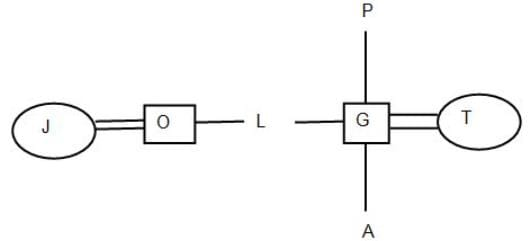Practice Test: Blood Relations - 1 - Question 7

Directions: Study the following information carefully to answer the given Questions:

P^Q-P is the child of Q
P!Q-P is the parent of Q
P*Q - P is elder to Q
P#Q-P is younger to Q
P@Q-P is brother of Q
P&Q - P is wife of Q
P+Q-P is sister-in-law of Q

Q. If A!B^C+D&E@F^GIA and G is the wife of H then how is G related to C?

Detailed Solution for Practice Test: Blood Relations - 1 - Question 7

A ! B ∧ C + D & E @ F ^ G! A: A is parent of B, B is child of C, C is sister-in-law of D, D is wife of E, E is brother of F, F is child of G, G is parent of A.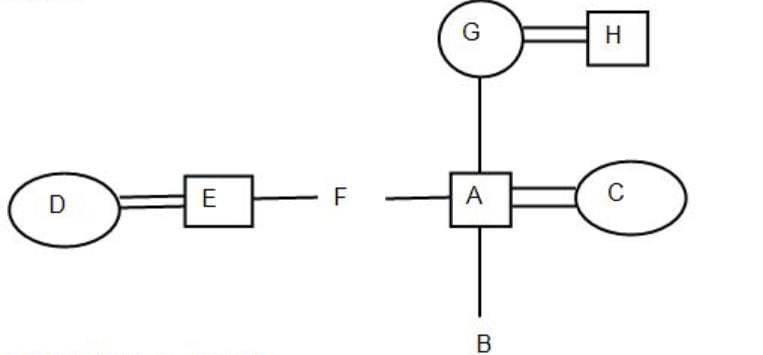Practice Test: Blood Relations - 1 - Question 8

Directions: Study the following information carefully to answer the given Questions:

P^Q-P is the child of Q
P!Q-P is the parent of Q
P*Q - P is elder to Q
P#Q-P is younger to Q
P@Q-P is brother of Q
P&Q - P is wife of Q
P+Q-P is sister-in-law of Q

Q. If A!B^C+D&E@F^G!A, if B is the son of C, then how is A related to B?

Detailed Solution for Practice Test: Blood Relations - 1 - Question 8

A ! B^C+D&E @F^G! A: A is parent of B, B is child of C, C is sister-in-law of D, D is wife of E, E is brother of F, F is child of G, G is parent of A. B is son of C.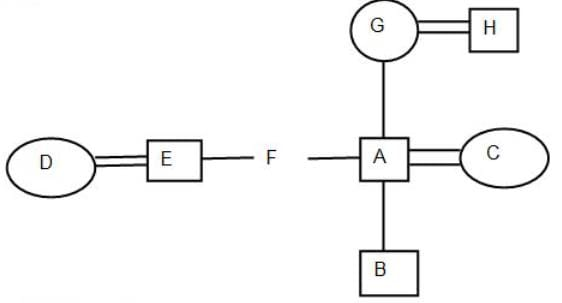Practice Test: Blood Relations - 1 - Question 9

Directions: Study the following information carefully to answer the given Questions:

P^Q-P is the child of Q
P!Q-P is the parent of Q
P*Q - P is elder to Q
P#Q-P is younger to Q
P@Q-P is brother of Q
P&Q - P is wife of Q
P+Q-P is sister-in-law of Q

Q. If P*Q^R@S#T*P, the age of Q is 22 years and age of T is 33 years, so what can be the age of P?

Detailed Solution for Practice Test: Blood Relations - 1 - Question 9

P*Q^R@S#T*P: P is elder than Q, Q is child of R, R is brother of S, S is younger than T, T is elder than P.
The age of Q is 22 years and age of T is 33 years.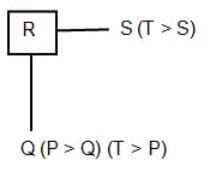T(33) > P > Q
Hence, the age of P is 29 years.

Practice Test: Blood Relations - 1 - Question 10

Directions: Study the following information carefully to answer the given Questions:

P^Q-P is the child of Q
P!Q-P is the parent of Q
P*Q - P is elder to Q
P#Q-P is younger to Q
P@Q-P is brother of Q
P&Q - P is wife of Q
P+Q-P is sister-in-law of Q

Q. If I!J@K#L^I!M*K, the age of M is 25 years and age of L is 32 years, so what can be the age of K?

## CSAT Preparation

197 videos|151 docs|200 tests
Information about Practice Test: Blood Relations - 1 Page
In this test you can find the Exam questions for Practice Test: Blood Relations - 1 solved & explained in the simplest way possible. Besides giving Questions and answers for Practice Test: Blood Relations - 1, EduRev gives you an ample number of Online tests for practice

## CSAT Preparation

197 videos|151 docs|200 tests

### How to Prepare for UPSC

Read our guide to prepare for UPSC which is created by Toppers & the best Teachers